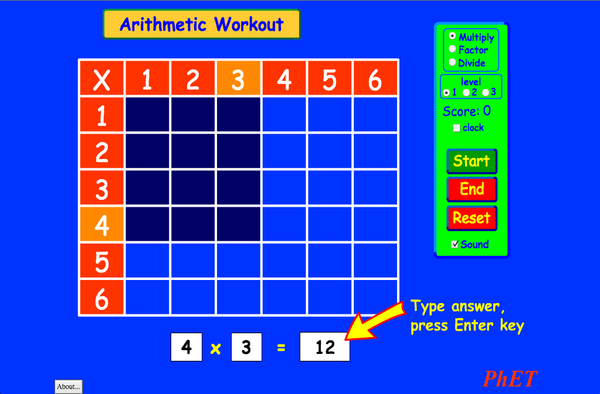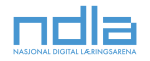# RechnenDownloadEinbinden schließen Einbinden einer Kopie dieser Simulation Verwenden Sie diese HTML-Datei um eine Kopie dieser Simulation einzubinden. Sie könenn die Breite und Höhe der eingebetteten Simulation durch Veränderung der Attribute "width" und "height" ändern. Einbinden eines Bildes, welches die Simulation beim Anklicken startet
Zum Starten klicken
Verwenden Sie diese HTML-Code um einen Screenshot mit den Worten "Zum Starten klicken" anzuzeigen. Multiplikation PhET wird unterstützt vonund Dozenten wie Ihnen.Zurück zur HTML5 Version

### Inhalt

• Multiplikation

### Beschreibung

Beherrschen Sie noch das Einmaleins? Frischen Sie Ihre Rechenfähigkeiten mit diesem spannenden Spiel auf (Multiplikation, Division und Faktorisieren).#
Keine Taschenrechner erlaubt!

### Lernziele

• Lernen Sie mit dem Einmaleins multiplizieren, dividieren und faktorisieren.
• Erhöhen Sie Ihre Genauigkeit beim Multiplizieren, Dividieren und Faktorisieren.

### Standard Alignment

#### Schulmathematik

3.OA.A.1
Interpret products of whole numbers, e.g., interpret 5 × 7 as the total number of objects in 5 groups of 7 objects each. For example, describe a context in which a total number of objects can be expressed as 5 × 7.
3.OA.A.2
Interpret whole-number quotients of whole numbers, e.g., interpret 56 ÷ 8 as the number of objects in each share when 56 objects are partitioned equally into 8 shares, or as a number of shares when 56 objects are partitioned into equal shares of 8 objects each. For example, describe a context in which a number of shares or a number of groups can be expressed as 56 ÷ 8.
3.OA.A.4
Determine the unknown whole number in a multiplication or division equation relating three whole numbers. For example, determine the unknown number that makes the equation true in each of the equations 8 × ? = 48, 5 = _ ÷ 3, 6 × 6 = ?
3.OA.B.5
Apply properties of operations as strategies to multiply and divide.2 Examples: If 6 × 4 = 24 is known, then 4 × 6 = 24 is also known. (Commutative property of multiplication.) 3 × 5 × 2 can be found by 3 × 5 = 15, then 15 × 2 = 30, or by 5 × 2 = 10, then 3 × 10 = 30. (Associative property of multiplication.) Knowing that 8 × 5 = 40 and 8 × 2 = 16, one can find 8 × 7 as 8 × (5 + 2) = (8 × 5) + (8 × 2) = 40 + 16 = 56. (Distributive property.)
3.OA.B.6
Understand division as an unknown-factor problem. For example, find 32 ÷ 8 by finding the number that makes 32 when multiplied by 8.
3.OA.C.7
Fluently multiply and divide within 100, using strategies such as the relationship between multiplication and division (e.g., knowing that 8 × 5 = 40, one knows 40 ÷ 5 = 8) or properties of operations. By the end of Grade 3, know from memory all products of two one-digit numbers.
3.OA.D.9
Identify arithmetic patterns (including patterns in the addition table or multiplication table), and explain them using properties of operations. For example, observe that 4 times a number is always even, and explain why 4 times a number can be decomposed into two equal addends.
Version 2.03

### Dozenten TippsÜberblick über Simulations Kontrolle, Modell Vereinfachung und Einsichten in Denkweise der Studenten ( PDF ).

### Aktivitäten, die von Dozenten eingereicht wurden

Juego de Aritmética (Basado en Indagación)Trish Loeblein, traducido por Diana López S II
S I
HA
Geführt
Mathematik
How do PhET simulations fit in my middle school program?Sarah Borenstein S I Sonst Geowissenschaften
Physik
Biologie
Chemie
MS and HS TEK to Sim Alignment Elyse Zimmer S II
S I
Sonst Chemie
Biologie
Physik
Math facts - Using the Multiplication Chart Tapan Sarkar S I Prakt Mathematik
Back to the Basics Nyetta Abernathy GS
S I
S II
Prakt
HA
Mathematik
Discovering divisibility rules Stacy Larson S I
S II
Prakt Mathematik
곱셈 나눗셈 연습 SIM 사용설명서 이화국(Wha Kuk Lee) S I
GS
KFn
HA
Demo
Physik
Mathematik
ARITHMETIC_DIVISON Arlete Cabral GS Prakt Mathematik
OBJETO DE APRENDIZAGEM: ARITHMETIC Arlete Moura de Oliveira Cabral e Maria Cleide da Silva Barroso GS Geführt Mathematik
Operações Aritméticas Fundamentais Georgyana Cidrão; Francisco Régis Vieira Alves GS Prakt
Geführt
Mathematik
Afrikaans Alle AfrikaansRekenkunde
Albanian Alle shqipAritmetika
Amharic Alle Amharicየሒሳብ ስሌቶች
Arabisch Alle العربيةarithmetic
Arabisch (Saudi Arabien) Alle العربية (السعودية)arithmetic
Basque Alle EuskaraAritmetika
Bosnian Alle BosanskiARITMETIKA
Chinesisch (traditionell) Alle 中文 (台灣)算數
Chinesisch (vereinfacht) Alle 中文 (中国)算术
Croatian Alle hrvatskiAritmetika
Danish Alle DanskAritmetisk
Englisch Alle EnglishArithmetic
Estonian Alle EestiAritmeetika
Französisch Alle françaisArithmétique
Georgian Alle ქართულიარითმეტიკა
Griechisch Alle ΕλληνικάΑριθμητικός
Hebrew Alle עבריתחשבון
Indonesian Alle Bahasa IndonesiaAritmatika
Italienisch Alle italianotabelline
Japanisch Alle 日本語計算
Kazakh Alle KazakhАрифметика
Korean Alle 한국어곱셈 나눗셈 연습
Latvian Alle LatviešuAritmētika
Macedonian Alle македонскиАРИТМЕТИКА
Marathi Alle मराठीअंकगणित
Niederländisch Alle NederlandsRekenen
Persian Alle فارسیحساب
Polish Alle polskiArytmetyka
Portugiesisch (Brasilien) Alle português (Brasil)Aritmética
Romanian Alle românăAritmetica
Russian Alle русскийАрифметика
Schwedisch Alle svenskaMatteräkning
Serbian Alle СрпскиАРИТМЕТИКА
Slovak Alle SlovenčinaAritmetika
Spanisch (Mexiko) Alle español (México)Aritmética
Spanisch (Peru) Alle español (Perú)Aritmética
Spanish Alle españolAritmética
Swahili Alle SwahiliHesabu
Türkisch Alle TürkçeAritmetik
Turkmen Alle TurkmenArifmetika
Ungarisch Alle magyarSzorzótábla játék
Vietnamese Alle Tiếng ViệtSố học
Windows Macintosh Linux
Microsoft Windows
XP/Vista/7/8.1/10
Macromedia Flash 9 or later
OS X 10.9.5 or later
Macromedia Flash 9 or later
Macromedia Flash 9 or later
Design Team Externe Quellen Dank an